Section2

# Leakage Detection Tutorial, Part 2: Detection on Real World Traces¶

In Part 1, we have introduced the t-test as methodology for leakage detection. Despite the simplicity of the procedure, we did not discuss about several practical relevant problems of detection that occur in the real world. In this part of the tutorial, we will discuss of the applicability of the t-test and the correlation-based test on real power traces. Datasets used in this Section can be downloaded at https://zenodo.org/record/2575405.

## Case study: AES-128 on a 8-bit microprocessor¶

As we have mentioned at the beginning of the previous part of this tutorial, a set of traces is collected stimulating a target device repeaditly, while monitoring its power consumption.

To investigate further the meaning of the lesson learned in the previous part, we will use real power traces collected on an unprotected AES-128 software implementation (AESFURIOUS by B. Poettering) on the popular platform ChipWhisperer-Lite from NewAE Technology. As device under test, we have used the on-board target, the 8bit microcontroller Atmel XMEGA128D4. The clock frequency has been set at 7.38MHz, while the sample rate has been set at 29.54MHz, which ensures 4 samples per clock cycle.

### Visual inspection of a power trace¶

The simplest approach to gain some useful information from the activity of the target device is to perform a visual inspection of a power trace, in order to spot some typical patterns, revealing the nature of the activity of the device itself. This basic analysis is also called Simple Power Analysis.

In :
import numpy as np
import matplotlib.pyplot as plt
import pandas as pd
from numpy import array
from IPython.display import display
from LUTs import HW_LUT, SBox_LUT

In :
#Loading the dataset:

#Extract the name of datafields:
data_fields = data.files
#Extract dimensions:
dim_fields = []
dtype_fields = []
for i in range(0, len(data.keys()),1):
dim_fields.append(data[data_fields[i]].shape)
dtype_fields.append(data[data_fields[i]].dtype)

indexlist = ['Rows,Columns', 'DataType']
df = pd.DataFrame(data=[dim_fields[:][:], dtype_fields], index=indexlist,
columns=data_fields)
display(df)

pt pt_fixed key ct traces flag meta
Rows,Columns (2000, 16) (1, 16) (1, 16) (2000, 16) (2000, 16000) (2000, 1) ()
DataType int32 int32 int32 int32 uint16 int32 object

Every dataset in this part of the tutorial contains the following data:

• traces: 10bit resolution raw traces, encoded in uint16;
• pt: 16byte plaintexts;
• ct: 16byte ciphertexts;
• key: 16byte key;
• flag (t-test datasets only): binary vector, 1 -> fixed class, 0 -> random class;
• meta: metadata associated to each file.

The matrix pt contains the input plaintext used during a specific experiement. Each row corresponds to a different query of the cryptographic algorithm, while columns corresponds to different bytes in a query. Similarly, ct represents the output ciphertexts and it is structured in the same way.Regarding _ptfixed, it represents the fixed-class plaintext using in TVLA experiments. The vector key containts the fixed key (the same in each experiment of the part). The flag binary vector express wheter the i-th query has been demanded with the fixed (flag=1) or the random class (flag=0). The matrix traces contains the raw traces digitalized at 10bit, thus encoded in np.uint16 for the sake of compactness. Each row contains a full trace of the AES-128, that is composed by 16000 samples.

In :
fignum=1
#Compute a mean trace:
MT = np.mean(data['traces'], axis=0)
Ntraces, Nsamples = data['traces'].shape

plt.figure(fignum)
plt.clf()
plt.plot(MT);
plt.xlabel('Time Samples');
plt.ylabel('Norm. Power');
plt.title('Figure %.0f: Atmel XMEGA128D4 - ChipWhisperer Lite' % fignum)
plt.plot([2500,2500],[50,800], ':k')
plt.plot([13900,13900],[50,800], ':k')
plt.xlim(0, Nsamples-1)
plt.show()
fignum = fignum + 1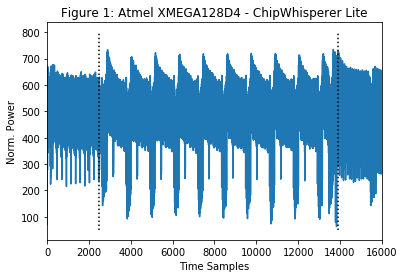As we can see, the power trace is mainly composed by three main parts: % block A, from sample 1 to sample ~2500; block B, from sample ~2500 to sample ~14000; * block C, from sample ~14000 to sample 16000.

• block A, from sample 1 to sample ~2500;
• block B, from sample ~2500 to sample ~13900;
• block C, from sample ~13900 to sample 16000.

Block A corresponds to the generatorion of the 10 round keys, computed offline in this implementation. Block C corresponds to the flushing operation of the ciphertext throughout the communication interface. Block B contains the power activity of the device while performing the AES-128 algorithm, and we are interested in understanding if we can detect or not any leakage in this time window. In fact, we can already learn something just by looking at the power trace: it is very easy (in this case) to note the presence of 10 identical patterns along block B. This behavior is typical of AES-128, which corresponds to 10 rounds of the algorithm.

In this part of the tutorial we will investigate the leakage detection only for the $1^{st}$ byte, without any loss of generality.

### Some definitions…¶

Before we go deeper in the analysis of real traces, we need to precisely assess some definitions, according to TVLA framework:

• Non-Specific test: it aims to detect any leakage that depends on input data (or key);
• Specific test: it tests target specific intermediate values of the cryptographic algorithm that could be exploited to recover keys or sensitive information.

## Fixed-versus-random T-test on real traces¶

The fixed-versus-random t-test is intrinsically a non-specific test, which can run very fast, since it is based on simple operations. Although, the “lightweight” property of the t-test has some drawbacks. As we have seen in Part 1, the t-test provides different detection rate depending on the chosen fixed class. In other words, the ability of the t-test to detect leakage relies heavily on the signal-to-noise ratio that the chosen fixed class can provide.

### Experiment 1¶

Let’s perform a first experiment with 2000 traces with a given fixed input $F^{1}$. Of course, we will have now real power traces to work on, so, we will perform the t-test on each time sample. In other words, the t-test in the standard TVLA is univariate and each time sample is considered as a single dimension.

In :
del data

#Casting raw samples in traces from uint16 to float:
traces = (data['traces']).astype(np.float)
#Extracting the flag vector:
flag = data['flag']
#Extracting the shape of the traces matrix:
Ntraces, Nsamples = traces.shape
#Index of the 'fixed plaintext' traces:
tF_index = flag==1;
#Index of the 'random plaintext' traces:
tR_index = flag==0;
#Number of queries with fixed class:
NF = tF_index.sum()
#Number of queries with random class:
NR = tR_index.sum()

#Sample mean of the 'fixed plaintext' traces
meanF = np.mean(traces[tF_index[:,0],:],axis=0)
#Sample mean of the 'random plaintext' traces
meanR = np.mean(traces[tR_index[:,0],:],axis=0)
#Sample variance of the 'fixed plaintext' traces
varF = np.var(traces[tF_index[:,0],:],axis=0)
#Sample variance of the 'random plaintext' traces
varR = np.var(traces[tR_index[:,0],:],axis=0)
#The formula for Welch's t-statistic:
tStatistic_F1  = (meanF - meanR)/np.sqrt(varF/NF + varR/NR)

#Indices of leaky samples (exceeding the threshold of +/-4.5):
threshold = np.abs(tStatistic_F1 [0:13900]) > 4.5

if threshold.any()==False:
print('No leakage detected.')
else:
ind_t = array(range(len(threshold)))
ind_t = ind_t[threshold]
if threshold.sum() == 1:
leak_str = 'Leakage detected in sample'
print(leak_str + ' %.0f.' % ind_t)
print('Total leaky sample: 1.')
else:
leak_str = 'Leakage detected in samples'
if len(ind_t) > 6:
for i in np.arange(6):
leak_str = leak_str + ' %.0f' % ind_t[i]
leak_str = leak_str + ' ...'
else:
for i in ind_t:
leak_str = leak_str + ' %.0f' % ind_t[i]
print(leak_str)
print('Total leaky points: %.0f.' % len(ind_t))

plt.figure(fignum)
plt.clf()
MT = np.mean(traces,axis=0)
plt.plot(MT)
plt.xlim(0, Nsamples-1)
plt.xlabel('Time Samples')
plt.ylabel('Norm. Power')
plt.title('Figure %.0f: Power trace (as reference)' % fignum)
fignum = fignum+1

plt.figure(fignum)
plt.clf()
plt.plot(tStatistic_F1)
plt.plot([0, Nsamples-1],[4.5, 4.5], '--r')
plt.plot([0, Nsamples-1],[-4.5, -4.5], '--r')
plt.xlim(0, Nsamples-1)
plt.xlabel('Time Samples')
plt.ylabel('t-statistic')
plt.title('Figure %.0f: Fixed vs Random Exp.1 (%.0f traces)' % (fignum, Ntraces))
plt.show()
fignum = fignum + 1

Leakage detected in samples 2505 2506 2507 2508 2513 2514 ...
Total leaky points: 9390.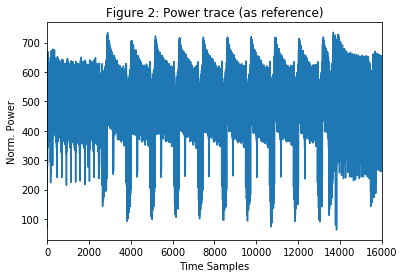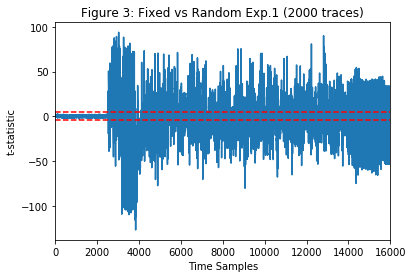From Part 1, we have learnt that if a point exceeds the +/-4.5 threshold in the t-test, it is considered as leaky. As we can observe from Figure 3, we have a large amount of leaky point! It is natural than to wonder why we have so much leaky points in the observed time window, and how we can get intuitions from this analysis from a security perspective.

The diffusion of AES will uniformly distribute intermediate values all over the state after a couple of rounds. This will provoke a strong diversification in the power consumption that will be detected by the test. The non-specific t-test will detect all points that depend on the input plaintext. This aspect is critical and reflects its non-specific nature. In fact, we did not fix any intermediate value (e.g. the output byte of the $1^{st}$ S-BOX at the first round), but only a fixed input plaintext has been used to perform the test. It has to be noted that no points have been detected in block A. As we have said before, the computation of the 10 round keys are computed off-line in this implementation of the AES which takes place exactly during block A. In our experiment, we have used the same key for all experiments. Thus, the power consumption of this section is the same for all traces, and no leaky points are detected in block A.

### Experiment 2¶

In this section, we will analyze a second set of traces for fixed-versus-random t-test, where the fixed class $F^{2}$ is different from Experiment 1.

In :
del data

#Casting raw samples in traces from uint16 to float:
traces = (data['traces']).astype(np.float)
#Extracting the flag vector:
flag = data['flag']
#Extracting the shape of the traces matrix:
Ntraces, Nsamples = traces.shape
#Index of the 'fixed plaintext' traces:
tF_index = flag==1;
#Index of the 'random plaintext' traces:
tR_index = flag==0;
#Number of queries with fixed class:
NF = tF_index.sum()
#Number of queries with random class:
NR = tR_index.sum()

#Sample mean of the 'fixed plaintext' traces
meanF = np.mean(traces[tF_index[:,0],:],axis=0)
#Sample mean of the 'random plaintext' traces
meanR = np.mean(traces[tR_index[:,0],:],axis=0)
#Sample variance of the 'fixed plaintext' traces
varF = np.var(traces[tF_index[:,0],:],axis=0)
#Sample variance of the 'random plaintext' traces
varR = np.var(traces[tR_index[:,0],:],axis=0)
#The formula for Welch's t-statistic:
tStatistic_F2  = (meanF - meanR)/np.sqrt(varF/NF + varR/NR)

#Indices of leaky samples (exceeding the threshold of +/-4.5):
threshold = np.abs(tStatistic_F2[0:13900]) > 4.5

if threshold.any()==False:
print('No leakage detected.')
else:
ind_t = array(range(len(threshold)))
ind_t = ind_t[threshold]
if threshold.sum() == 1:
leak_str = 'Leakage detected in sample'
print(leak_str + ' %.0f.' % ind_t)
print('Total leaky sample: 1.')
else:
leak_str = 'Leakage detected in samples'
if len(ind_t) > 6:
for i in np.arange(6):
leak_str = leak_str + ' %.0f' % ind_t[i]
leak_str = leak_str + ' ...'
else:
for i in ind_t:
leak_str = leak_str + ' %.0f' % ind_t[i]
print(leak_str)
print('Total leaky points: %.0f.' % len(ind_t))

plt.figure(fignum)
plt.clf()
plt.plot(tStatistic_F2)
plt.plot([0, Nsamples-1],[4.5, 4.5], '--r')
plt.plot([0, Nsamples-1],[-4.5, -4.5], '--r')
plt.xlim(0, Nsamples-1)
plt.xlabel('Time Samples')
plt.ylabel('t-statistic')
plt.title('Figure %.0f: Fixed vs Random Exp.2 (%.0f traces)' % (fignum, Ntraces))
plt.show()
fignum = fignum + 1

Leakage detected in samples 2505 2506 2507 2508 2509 2510 ...
Total leaky points: 9422.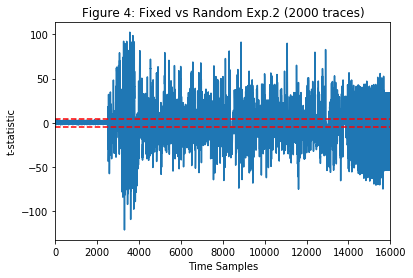Also in this case, we detected a large amount of leaky points. It is easy to observe that the number of detected points is slightly different.

In :
plt.figure(fignum)
plt.clf()
plt.plot(tStatistic_F1,label='Experiment 1')
plt.plot(tStatistic_F2,'k',label='Experiment 2')
plt.plot([0, Nsamples-1],[4.5, 4.5], '--r')
plt.plot([0, Nsamples-1],[-4.5, -4.5], '--r')
plt.xlim((2500, 3500))
plt.xlabel('Time Samples')
plt.ylabel('t-statistic')
plt.legend()
plt.title('Figure %.0f: Zoom on Fixed vs Random on two different fixed classes (%.0f traces)' % (fignum, Ntraces))
fignum = fignum + 1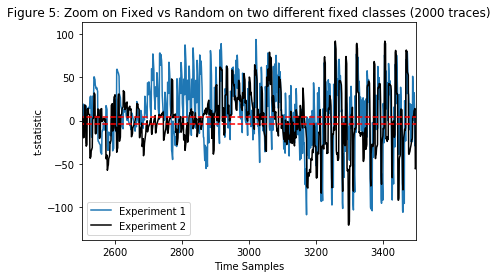It is clear that the two experiments will not provide the same outcome even in terms of which point is leaking. In Figure 5, we can observe that Experiment 1 and Experiment 2 are not overlapping perfectly. This effect is due to the difference in the chosen fixed class. In fact, choosing different fixed class will mean (in general) that the power consumption for the fixed class’ traces will be different from the previous experiment, and thus, the t-test will not detect the same points. The fixed-versus-random non-specific t-test in TVLA is able to check if an implementation is leaking or not, but it will not be able to provide more information on the nature of the leakage.

It is interesting to check if we can get detection with a reduced number of set. In the following experiment, we have reduced the number of traces from 2000 to 50 on the dataset collected with $F^{2}$.

In :
del data

#Sample size:
samplesize = 50

#Casting raw samples in traces from uint16 to float:
traces = (data['traces']).astype(np.float)
#Extracting the flag vector:
flag = data['flag']

#Reduce the number of traces used for detection
traces = traces[0:samplesize,:]
flag = flag[0:samplesize,:]

#Extracting the shape of the traces matrix:
Ntraces, Nsamples = traces.shape

#Index of the 'fixed plaintext' traces:
tF_index = flag==1;
#Index of the 'random plaintext' traces:
tR_index = flag==0;
#Number of queries with fixed class:
NF = tF_index.sum()
#Number of queries with random class:
NR = tR_index.sum()

#Sample mean of the 'fixed plaintext' traces
meanF = np.mean(traces[tF_index[:,0],:],axis=0)
#Sample mean of the 'random plaintext' traces
meanR = np.mean(traces[tR_index[:,0],:],axis=0)
#Sample variance of the 'fixed plaintext' traces
varF = np.var(traces[tF_index[:,0],:],axis=0)
#Sample variance of the 'random plaintext' traces
varR = np.var(traces[tR_index[:,0],:],axis=0)
#The formula for Welch's t-statistic:
tStatistic_F2R  = (meanF - meanR)/np.sqrt(varF/NF + varR/NR)

#Indices of leaky samples (exceeding the threshold of +/-4.5):
threshold = np.abs(tStatistic_F2R [0:13900]) > 4.5

if threshold.any()==False:
print('No leakage detected.')
else:
ind_t = array(range(len(threshold)))
ind_t = ind_t[threshold]
if threshold.sum() == 1:
leak_str = 'Leakage detected in sample'
print(leak_str + ' %.0f.' % ind_t)
print('Total leaky sample: 1.')
else:
leak_str = 'Leakage detected in samples'
if len(ind_t) > 6:
for i in np.arange(6):
leak_str = leak_str + ' %.0f' % ind_t[i]
leak_str = leak_str + ' ...'
else:
for i in ind_t:
leak_str = leak_str + ' %.0f' % ind_t[i]
print(leak_str)
print('Total leaky points: %.0f.' % len(ind_t))

plt.figure(fignum)
plt.clf()
plt.plot(tStatistic_F2R )
plt.plot([0, Nsamples-1],[4.5, 4.5], '--r')
plt.plot([0, Nsamples-1],[-4.5, -4.5], '--r')
plt.xlim(0, Nsamples-1)
plt.xlabel('Time Samples')
plt.ylabel('t-statistic')
plt.title('Figure %.0f: Fixed-versus-random t-test Exp.2 (%.0f traces)' % (fignum, Ntraces))
plt.show()
fignum = fignum + 1

Leakage detected in samples 2508 2514 2515 2525 2526 2527 ...
Total leaky points: 2734.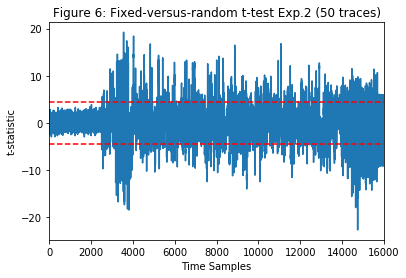The maximum value of the t-statistic is, of course, lower, since the number of traces for the detection is smaller, but we can still observe (with sufficient confidence) approximately the same number of leaky points as for the full dataset above. It is straightforward to conclude that in this case, the false positive over the time window is very high (probably not all detected points are really leaky or exploitable), and we cannot argue when a leaky point is really leaking information!

## Fixed-versus-Fixed T-test¶

The fixed-versus-random t-test in the TVLA procedure is non-specific, so it will be able to detect all the differences that propagate through the trace based on the choice of fixed input class. It will not be able to focalize the detection of a specific (and possibly exploitable) intermediate function of the algorithm, since the partition is simply done on two input classes, regardless of intermediate results at any poiny in time. As we have seen in previous experiments, the fixed-versus-random non-specific t-test is not suitable to make a selection of points-of-interest (POIs), especially for software implementation, as in our case. The high number of false positive along the time window will not help in reducing the data complexity for attacking the device, regarding DPA/CPA procedures.

In order to increase the efficiency of the detection, and attacker/evaluator can use fixed-versus-fixed t-test for leakage detection is a testing procedure which is very similar to the fixed-versus-random. They share the same procedure and, basically, no additional operations are required. In this case, the two classes used for testing are both fixed, and, as we will explain later, it is preferable to the classic fixed-versus-random since it allows (in general) larger differences between the two classes. In the definition of the signal-to-noise ratio in “Hardware Countermeasure against DPA – A Statistical Analysis of Their Effectiveness” by S.Mangard (CT-RSA 2004), the noise component of a t-test trace in the fixed-versus-random scenario is due to the sum of the physical noise ($2\sigma_{n}$) and the algorithmic noise ($\sigma_{alg}$). The latter one comes from the variation due to the random class, which in practice, can be seen as noise. The differential nature of the fixed-versus-fixed t-test will reduce the overall noise to $2\sigma_{n}$, since the algorithmic noise will not contribute. We can observe this practical evidence by looking at histograms from a fixed-versus-fixed against a fixed-verus-random experiment.

In :
#Time sample for histogram investigation
sample = 2960

#Casting raw samples in traces from uint16 to float:
traces = (data['traces']).astype(np.float)
#Extracting the flag vector:
flag = data['flag']

#Index of the class A traces:
tA_index = flag==1
#Index of the class B traces:
tB_index = flag==0

plt.figure(fignum)
plt.hist(traces[tA_index[:,0],sample],bins=50,histtype='bar',label='Class A')
plt.hist(traces[tB_index[:,0],sample],bins=50,histtype='bar',label='Class B')
plt.xlabel('Norm. Power')
plt.ylabel('Occurencies')
plt.legend()
plt.title('Figure %.0f: Fixed-versus-fixed' % fignum)
plt.show()
fignum = fignum + 1

#Casting raw samples in traces from uint16 to float:
traces = (data['traces']).astype(np.float)
#Extracting the flag vector:
flag = data['flag']

#Index of the fixed class traces:
tF_index = flag==1
#Index of the random class traces:
tR_index = flag==0

plt.figure(fignum)
plt.clf()
plt.hist(traces[tF_index[:,0],sample],bins=50,histtype='bar',label='Fixed Class')
plt.hist(traces[tR_index[:,0],sample],bins=50,histtype='bar',label='Random Class')
plt.xlabel('Norm. Power')
plt.ylabel('Occurencies')
plt.legend()
plt.title('Figure %.0f: Fixed-versus-random' % fignum)
plt.show()
fignum = fignum + 1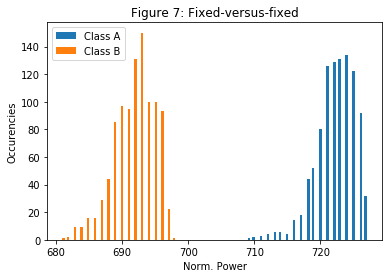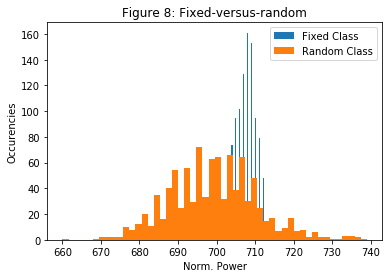It is clear that in the fixed-versus-fixed, the separation between the two class is larger, and it makes easier to detect this leakage. In the fixed-versus-random experiment, the variance of the random class is very large, and its distribution of the power consumption strongly overlap with the distribution of the fixed class.

### Experiment 1¶

We want to investigate the outcome of the fixed-versus-fixed test on the whole time window, with the same number of traces used in Experiment 1 and 2.

In :
del data

#Casting raw samples in traces from uint16 to float:
traces = (data['traces']).astype(np.float)
#Extracting the flag vector:
flag = data['flag']

#Number of traces/queries to use in the experiment:
Ntraces = 2000
traces = traces[0:Ntraces,:]
flag = flag[0:Ntraces]

#Extracting the number of samples per trace:
Nsamples = traces.shape

#Index of the 'fixed A plaintext' traces:
tA_index = flag==1;
#Index of the 'fixed B plaintext' traces:
tB_index = flag==0;
#Number of queries with fixed A class:
NA = tA_index.sum()
#Number of queries with fixed B class:
NB = tB_index.sum()

#Sample mean of the 'fixed A plaintext' traces
meanA = np.mean(traces[tA_index[:,0],:],axis=0)
#Sample mean of the 'fixed B plaintext' traces
meanB = np.mean(traces[tB_index[:,0],:],axis=0)
#Sample variance of the 'fixed A plaintext' traces
varA = np.var(traces[tA_index[:,0],:],axis=0)
#Sample variance of the 'fixed B plaintext' traces
varB = np.var(traces[tB_index[:,0],:],axis=0)
#The formula for Welch's t-statistic:
tStatistic_FvF  = (meanA - meanB)/np.sqrt(varA/NA + varB/NB)

#Indices of leaky samples (exceeding the threshold of +/-4.5):
threshold = np.abs(tStatistic_FvF [0:13900]) > 4.5

if threshold.any()==False:
print('No leakage detected.')
else:
ind_t = array(range(len(threshold)))
ind_t = ind_t[threshold]
if threshold.sum() == 1:
leak_str = 'Leakage detected in sample'
print(leak_str + ' %.0f.' % ind_t)
print('Total leaky sample: 1.')
else:
leak_str = 'Leakage detected in samples'
if len(ind_t) > 6:
for i in np.arange(6):
leak_str = leak_str + ' %.0f' % ind_t[i]
leak_str = leak_str + ' ...'
else:
for i in ind_t:
leak_str = leak_str + ' %.0f' % ind_t[i]
print(leak_str)
print('Total leaky points: %.0f.' % len(ind_t))

plt.figure(fignum)
plt.clf()
plt.subplot(211)
plt.plot(tStatistic_FvF )
plt.plot([0, Nsamples-1],[4.5, 4.5], '--r')
plt.plot([0, Nsamples-1],[-4.5, -4.5], '--r')
plt.xlim(0, Nsamples-1)
#Vertical zoom on the processing part
maxAbsT = np.max(np.abs(tStatistic_FvF [0:13900]))+0.2*np.max(np.abs(tStatistic_FvF [0:13900]));
plt.ylim(-maxAbsT, maxAbsT)
plt.xlabel('Time Samples')
plt.ylabel('t-statistic')
plt.title(r'Figure %.0f: Fixed vs Fixed Exp.1 (%.0f traces)' % (fignum, Ntraces))
plt.show()
plt.subplot(212)
plt.plot(tStatistic_FvF)
plt.plot([0, Nsamples-1],[4.5, 4.5], '--r')
plt.plot([0, Nsamples-1],[-4.5, -4.5], '--r')
plt.xlim(2000,4000)
maxAbsT = np.max(np.abs(tStatistic_FvF[2000:4000]))+0.2*np.max(np.abs(tStatistic_FvF[2000:4000]));
plt.ylim(-maxAbsT, maxAbsT)
plt.xlabel('Time Samples')
plt.ylabel('t-statistic')
plt.title('Zoom Samples Interval (2000;4000)')
plt.show()
fignum = fignum + 1

Leakage detected in samples 54 55 56 85 86 2509 ...
Total leaky points: 10214.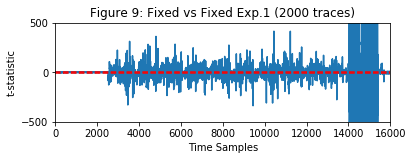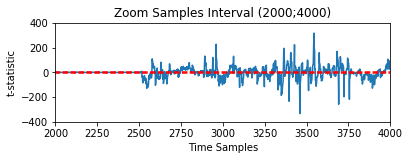From Figure 9 we can observe that we have reached two times the t-statistic with the same sample size. Also in this case, the diffusion tends to highlight a large number of leaky points after the first round.

### Experiment 2¶

In Experiment 2, we have chosen two different input classes, performing again the fixed-versus-fixed t-test.

In :
del data

#Casting raw samples in traces from uint16 to float:
traces = (data['traces']).astype(np.float)
#Extracting the flag vector:
flag = data['flag']

#Number of traces/queries to use in the experiment:
Ntraces = 2000
traces = traces[0:Ntraces,:]
flag = flag[0:Ntraces]

#Extracting the number of samples per trace:
Nsamples = traces.shape

#Index of the 'fixed A plaintext' traces:
tA_index = flag==1;
#Index of the 'fixed B plaintext' traces:
tB_index = flag==0;
#Number of queries with fixed A class:
NA = tA_index.sum()
#Number of queries with fixed B class:
NB = tB_index.sum()

#Sample mean of the 'fixed A plaintext' traces
meanA = np.mean(traces[tA_index[:,0],:],axis=0)
#Sample mean of the 'fixed B plaintext' traces
meanB = np.mean(traces[tB_index[:,0],:],axis=0)
#Sample variance of the 'fixed A plaintext' traces
varA = np.var(traces[tA_index[:,0],:],axis=0)
#Sample variance of the 'fixed B plaintext' traces
varB = np.var(traces[tB_index[:,0],:],axis=0)
#The formula for Welch's t-statistic:
tStatistic_FvF2  = (meanA - meanB)/np.sqrt(varA/NA + varB/NB)

#Indices of leaky samples (exceeding the threshold of +/-4.5):
threshold = np.abs(tStatistic_FvF2 [0:13900]) > 4.5

if threshold.any()==False:
print('No leakage detected.')
else:
ind_t = array(range(len(threshold)))
ind_t = ind_t[threshold]
if threshold.sum() == 1:
leak_str = 'Leakage detected in sample'
print(leak_str + ' %.0f.' % ind_t)
print('Total leaky sample: 1.')
else:
leak_str = 'Leakage detected in samples'
if len(ind_t) > 6:
for i in np.arange(6):
leak_str = leak_str + ' %.0f' % ind_t[i]
leak_str = leak_str + ' ...'
else:
for i in ind_t:
leak_str = leak_str + ' %.0f' % ind_t[i]
print(leak_str)
print('Total leaky points: %.0f.' % len(ind_t))

plt.figure(fignum)
plt.clf()
plt.plot(tStatistic_FvF2 )
plt.plot([0, Nsamples-1],[4.5, 4.5], '--r')
plt.plot([0, Nsamples-1],[-4.5, -4.5], '--r')
plt.xlim(0, Nsamples-1)
#Vertical zoom on the processing part
maxAbsT = np.max(np.abs(tStatistic_FvF2 [0:13900]))+0.2*np.max(np.abs(tStatistic_FvF2 [0:13900]));
plt.ylim(-maxAbsT, maxAbsT)
plt.xlabel('Time Samples')
plt.ylabel('t-statistic')
plt.title(r'Figure %.0f: Fixed vs Fixed Exp.2 (%.0f traces)' % (fignum, Ntraces))
plt.show()
fignum = fignum + 1

plt.figure(fignum)
plt.clf()
plt.plot(tStatistic_FvF, label='Experiment 1')
plt.plot(tStatistic_FvF2, label='Experiment 2')
plt.plot([0, Nsamples-1],[4.5, 4.5], '--r')
plt.plot([0, Nsamples-1],[-4.5, -4.5], '--r')
plt.xlim(2000,4000)
maxAbsT = np.max(np.abs(tStatistic_FvF[2000:4000]))+0.2*np.max(np.abs(tStatistic_FvF[2000:4000]));
plt.ylim(-maxAbsT, maxAbsT)
plt.xlabel('Time Samples')
plt.ylabel('t-statistic')
plt.legend()
plt.title('Figure %.0f: Zoom Samples Interval (2000;4000)' % fignum)
plt.show()
fignum = fignum + 1

Leakage detected in samples 2505 2506 2507 2508 2509 2510 ...
Total leaky points: 10239.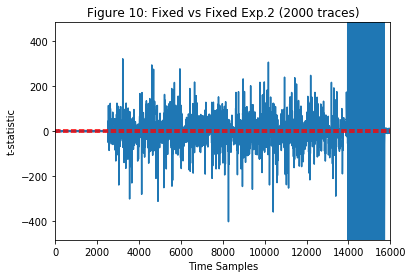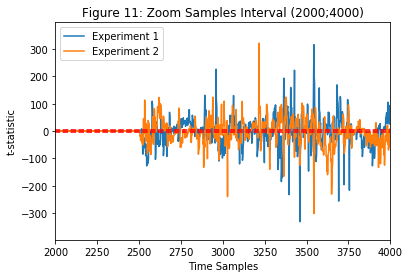Also in this case, the two experiments, performed with two different pairs of fixed classes, give different results.

## Correlation test, or $\rho$-test¶

The t-test procedure in the TVLA provides a useful tool for fast leakage detection, since it has a very low sampling complexity. On the other hand, the t-test in the TVLA is not able to provide useful information about leaky points over a long time window. In fact, it is often considered a pass/fail test for evaluating the presence of some data-dependency. Even under the perspective of detecting POIs to reduce the attack complexity, the t-test could not be the best choice. As we already mentioned in Part 1, we can make use of the correlation-based test, as known also as $\rho$-test, as proposed in “From Improved Leakage Detection to the Detection of Points of Interests in Leakage Traces” by Durvaux and Standaert at Eurocrypt’16. In this case, we will go a little bit more deeper than the previous part, introducing more in detail the $\rho$-test procedure. Instead of testing difference of means in the power consumption (it can be extended to side-channels, e.g. electromagnetic emission) between two classes, the $\rho$-test looks into correlation between measured power consumption and an estimated power model, in general, based on measurements. As we will see, this test will require a two-step procedure. In addition, the $\rho$-test makes use of a k-fold cross-validation technique to overcome bias in the statistical test. For a k-fold cross-validation, the collected traces L are split into k non overlapping sets $L^{i}$ of approximately the same size. We define profiling sets as:

$$L_{p}^{j} = \cup_{i \neq j} L^{i}$$

and test sets as:

$$L_{t}^{j} = L \setminus L_{p}^{j}$$

For each cross-validation set j ($1 \leq j \leq k$), a model is
estimated:

$$\hat{model}_{\tau}^{j} (X) \leftarrow L_{p}^{j}$$

where X is the input plaintext byte. The model is computed as the mean of
the partition of X, as reported in the following:

$$\hat{\mu}_{X}^{j}(t)$$

At this point, the Pearson’s correlation coefficient between the profiles
in $L_{p}^{j}$ and the test set $L_{t}^{j}$:

$$\hat{r}^{j}(\tau) = \hat{\rho}(L_{X}^{j},\hat{model}_{\tau}^{j}(X))$$

Of course, the correlation coefficient is computed sample-wise for all k
folds. The k correlation curves in time $\hat{r}^{j}(\tau)$ we have
obtained are then averaged to get a single result $\hat{r}(\tau)$,
representative for the whole dataset. As we already reported in Part 1, a
normalized Fisher’s z-transformation is then applied to correlation
coefficients.

A popular choice for the k parameter for cross-validation is 10 (see “An
Introduction to Statistical Learning” by James et al.), which ensure a
good trade-off between bias and variance of the test.

In :
#Loading data-set:

#In this case, the dataset we loaded does not contain _pt_fixed_ and _flag_, since

#Cast raw samples in traces from uint16 to float:
traces = (data['traces']).astype(np.float)
# Extract plaintexts from the dataset:
pt = data['pt']

#Delete unnecessary variables
del data

#Extracting the shape of the traces matrix:
Ntraces, Nsamples = traces.shape


In this case, the dataset we loaded does not contain pt_fixed and
flag, since they are not needed in this context.

In :
#Number of folds:
k = 10
#Byte to focus on (1st -> 0):
Byte_to_focus = 0

# *Folds allocation*

#Number of traces per set:
step = int(np.floor(Ntraces/k))
#Preallocate memory for profile sets and test sets (traces and plaintexts)
Lt = [None]*(k+k)
Lp = [None]*(k+k)

#Initialise sets to zeros
for i in range(0, 2*k, 1):
if np.mod(i,2) == 0:
#Lp[i] and Lt[i] with even index i will contain traces:
Lp[i] = np.zeros(((k-1)*step, Nsamples))
Lt[i] = np.zeros((step, Nsamples))
else:
#Lp[i] and Lt[i] with odd index i will contain traces:
Lp[i] = np.zeros(((k-1)*step,16), dtype=np.int)
Lt[i] = np.zeros((step,16), dtype=np.int)

t = 0
for i in range(0, k, 1):
q_in_Lp = 0
for j in range(0, k, 1):
if i != j:
#Profile sets:
Lp[t][q_in_Lp*step:(q_in_Lp+1)*step,:] = traces[j*step:(j+1)*step,:]
Lp[t+1][q_in_Lp*step:(q_in_Lp+1)*step,:] = pt[j*step:(j+1)*step,:]
q_in_Lp = q_in_Lp + 1
else:
#Test sets:
Lt[t] = traces[j*step:(j+1)*step,:]
Lt[t+1] = pt[j*step:(j+1)*step,:]
t = t + 2

# *Profiling phase*

#Number of traces in a profile set:
Ntraces_in_Lp = len(Lp[:,0])

#Initialise the matrix with profiles:
mu = [None]*k

t = 0
for j in range(0, k, 1):
#Temporary Profile set (traces and plaintext)
Lj = Lp[t]
Pj = Lp[t+1]

to_be_put_in_mu = np.zeros((256, Nsamples))

#Compute the mean of j-th profile set:
for i in range(0, 256, 1):
to_be_put_in_mu [i,:] =  np.mean(Lj[Pj[:,Byte_to_focus] == i, :],axis=0)

mu[j] = to_be_put_in_mu

t = t + 2

# *Detection phase*

Ntraces_in_Lt = Lt.shape
rhoj = np.zeros((k,Nsamples))

for j in range(0, k, 1):
#Temporary variables for test set (traces and plaintexts) and profiles
Ltj = Lt[j*2]
muj = mu[j]
#Efficient computation of correlation coefficient
Ltj_n =Ltj - np.mean(Ltj,axis=0)
Ltj_n = Ltj_n/np.sqrt(np.sum(np.square(Ltj_n),axis=0))
muj_n = muj - muj.mean(axis=0)
muj_n = muj_n/np.sqrt(np.sum(np.square(muj_n),axis=0))
rhoj[j,:] = np.sum(np.multiply(Ltj_n,muj_n), axis=0)

#The overall correlation coefficient is computed as the average accross the k
#folds
rho_total = np.mean(rhoj,axis=0)
#Fisher's z-transformation
rho_normalized = np.log((rho_total + 1)/ (- rho_total + 1))*np.sqrt(Ntraces_in_Lt - 3)*0.5

#Indices of leaky samples, exceeding the threshold of +/-4.5
threshold = np.abs(rho_normalized) > 4.5

if threshold.any()==False:
print('No leakage detected.')
else:
ind_t = array(range(len(threshold)))
ind_t = ind_t[threshold]
if threshold.sum() == 1:
leak_str = 'Leakage detected in sample'
print(leak_str + ' %.0f.' % ind_t)
print('Total leaky sample: 1.')
else:
leak_str = 'Leakage detected in samples'
if len(ind_t) > 6:
for i in np.arange(6):
leak_str = leak_str + ' %.0f' % ind_t[i]
leak_str = leak_str + ' ...'
else:
for i in ind_t:
leak_str = leak_str + ' %.0f' % ind_t[i]
print(leak_str)
print('Total leaky points: %.0f.' % len(ind_t))

plt.figure(fignum)
plt.clf()
plt.subplot(311)
plt.plot(muj[0,:])
if threshold.any() == True:
plt.plot(ind_t, muj[0,ind_t], 'r*', label='Leaky sample')
plt.legend(loc='upper right')
plt.xlabel('Time Samples')
plt.ylabel('Norm. Power')
plt.title('Figure %.0f: Leaky samples' % fignum )
plt.show()
plt.subplot(312)
plt.plot(rho_normalized)
plt.plot([0, Nsamples-1],[4.5, 4.5], '--r')
plt.plot([0, Nsamples-1],[-4.5, -4.5], '--r')
plt.xlim(0, Nsamples-1)
plt.xlim(0, Nsamples-1)
plt.xlabel('Time Samples')
plt.ylabel(r'$\hat{\rho}_{z}$')
plt.title('Correlation-test using input plaintext (%.0f traces)' % Ntraces)
plt.show()
plt.subplot(313)
plt.plot(rho_normalized)
plt.plot([0, Nsamples-1],[4.5, 4.5], '--r')
plt.plot([0, Nsamples-1],[-4.5, -4.5], '--r')
plt.xlim(0, Nsamples-1)
plt.xlim(2000, 4000)
plt.xlabel('Time Samples')
plt.ylabel(r'$\hat{\rho}_{z}$')
plt.title('Zoom Samples Interval (2000;4000)')
plt.show()
fignum = fignum + 1

Leakage detected in samples 2505 2506 2507 2508 2509 2510 ...
Total leaky points: 62.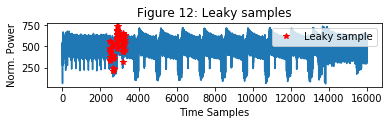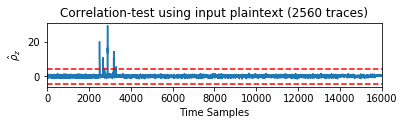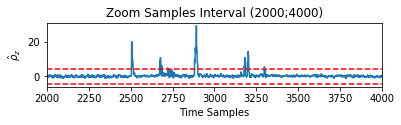It is clear that the number of leaky points is drastically decreased, and the remaining ones are concentrated at the beginning of the processing, that is, in other words, in the first round. These peaks are observed whenever the input plaintext byte, used for profiling, is manipulated bijectively with the fixed key (input loading, XOR and SBox). We must notice that the test as it has been presented it has to be considered non-specific, since we are only providing random plaintext and without targeting a specific intermediate value nor assuming a specific power model.

Compared to the t-test experiments, the output statistic of the $\rho$-test is much lower. In fact, the $\rho$-test requires higher sampling complexity (and computational power) compared to the t-test. The detection performed with this correlation-based test makes use of a large number of classes (that for the AES-128 is very handy to be 256) and provides useful POIs that are vulnerable with some specific attacks (e.g. CPA).

### Correlation-test with Hamming Weight Partitioning¶

We can improve also the partitioning of the $\rho$-test, in the direction of saving memory and computational complexity. Following the methodology used in the CPA, we can create a reduced model, based on the classic Hamming Weight partitioning of an intermediate value. We have used the output of the S-box of the $1^{st}$ byte at the first round. Of course, we are leveraging on strong assumptions:

• the device leaks following the Hamming Weight for this specific value;
• knowledge of the key (which is, in general, true for evaluation purpose).

Since we are focalizing the detection on a specific value, this test is specific. In this case, the profile set is used to derive 9 models, one per each value of the Hamming Weight of target intermediate result (the output of the 8bit S-box of the AES in this case).

In :
#Loading data-set:

#In this case, the dataset we loaded does not contain _pt_fixed_ and _flag_, since

#Cast raw samples in traces from uint16 to float:
traces = (data['traces']).astype(np.float)
#Extract plaintexts from the dataset:
pt = data['pt']
#Extract the key from the dataset:
key = data['key']

#Extracting the shape of the traces matrix:
Ntraces, Nsamples = traces.shape

#Number of folds:
k = 10
#Byte to focus on (1st -> 0):
Byte_to_focus = 0

# *Folds allocation*

#Number of traces per set:
step = int(np.floor(Ntraces/k))
#Preallocate memory for profile sets and test sets (traces and plaintexts)
Lt = [None]*(k+k)
Lp = [None]*(k+k)

#Initialise sets to zeros
for i in range(0, 2*k, 1):
if np.mod(i,2) == 0:
#Lp[i] and Lt[i] with even index i will contain traces:
Lp[i] = np.zeros(((k-1)*step, Nsamples))
Lt[i] = np.zeros((step, Nsamples))
else:
#Lp[i] and Lt[i] with odd index i will contain traces:
Lp[i] = np.zeros(((k-1)*step,16), dtype=np.int)
Lt[i] = np.zeros((step,16), dtype=np.int)

t = 0
for i in range(0, k, 1):
q_in_Lp = 0
for j in range(0, k, 1):
if i != j:
#Profile sets:
Lp[t][q_in_Lp*step:(q_in_Lp+1)*step,:] = traces[j*step:(j+1)*step,:]
Lp[t+1][q_in_Lp*step:(q_in_Lp+1)*step,:] = pt[j*step:(j+1)*step,:]
q_in_Lp = q_in_Lp + 1
else:
#Test sets:
Lt[t] = traces[j*step:(j+1)*step,:]
Lt[t+1] = pt[j*step:(j+1)*step,:]
t = t + 2

# *Profiling phase*

#Number of traces in a profile set:
Ntraces_in_Lp = len(Lp[:,0])

#Initialise the matrix with profiles:
mu = [None]*k

t = 0
for j in range(0, k, 1):
#Temporary Profile set (traces and plaintext)
Lj = Lp[t]
Pj = Lp[t+1]

to_be_put_in_mu = np.zeros((9, Nsamples))

#Compute the mean of j-th profile set:
for i in range(0, 9, 1):
to_be_put_in_mu [i,:] =  np.mean(Lj[HW_LUT(SBox_LUT(np.bitwise_xor(Pj[:,Byte_to_focus], key[0,Byte_to_focus]) ))== i, :],axis=0)

mu[j] = to_be_put_in_mu

t = t + 2

Ntraces_in_Lt = Lt.shape
rhoj = np.zeros((k,Nsamples))

for j in range(0, k, 1):
#Temporary variables for test set (traces and plaintexts) and profiles
Ltj = Lt[j*2]
Ptj = Lt[j*2+1]
muj = mu[j]
model_mat = np.zeros(Ltj.shape)
for i in range(0, Ntraces_in_Lt,1):
model_mat[i,:] = muj[HW_LUT(SBox_LUT(np.bitwise_xor(Pj[i,Byte_to_focus],
key[0,Byte_to_focus]) ))]

#Efficient computation of correlation coefficient
Ltj_n =Ltj - np.mean(Ltj,axis=0)
Ltj_n = Ltj_n/np.sqrt(np.sum(np.square(Ltj_n),axis=0))
model_mat_n = model_mat - model_mat.mean(axis=0)
model_mat_n = model_mat_n/np.sqrt(np.sum(np.square(model_mat_n),axis=0))
rhoj[j,:] = np.sum(np.multiply(Ltj_n,model_mat_n), axis=0)

#The overall correlation coefficient is computed as the average accross the k
#folds
rho_total = np.mean(rhoj,axis=0)
#Fisher's z-transformation
rho_normalized_HW = np.log((rho_total + 1)/ (- rho_total + 1))*np.sqrt(Ntraces_in_Lt - 3)*0.5

#Indices of leaky samples, exceeding the threshold of +/-4.5
threshold = np.abs(rho_normalized_HW) > 4.5

if threshold.any()==False:
print('No leakage detected.')
else:
ind_t = array(range(len(threshold)))
ind_t = ind_t[threshold]
if threshold.sum() == 1:
leak_str = 'Leakage detected in sample'
print(leak_str + ' %.0f.' % ind_t)
print('Total leaky sample: 1.')
else:
leak_str = 'Leakage detected in samples'
if len(ind_t) > 6:
for i in np.arange(6):
leak_str = leak_str + ' %.0f' % ind_t[i]
leak_str = leak_str + ' ...'
else:
for i in ind_t:
leak_str = leak_str + ' %.0f' % ind_t[i]
print(leak_str)
print('Total leaky points: %.0f.' % len(ind_t))

plt.figure(fignum)
plt.clf()
plt.subplot(211)
plt.plot(muj[0,:])
if threshold.any() == True:
plt.plot(ind_t, muj[0,ind_t], 'r*', label='Leaky sample')
plt.legend(loc='upper right')
plt.xlabel('Time Samples')
plt.ylabel('Norm. Power')
plt.title('Figure %.0f: Leaky samples' % fignum )
plt.show()
plt.subplot(212)
plt.plot(rho_normalized_HW)
plt.plot([0, Nsamples-1],[4.5, 4.5], '--r')
plt.plot([0, Nsamples-1],[-4.5, -4.5], '--r')
plt.xlim(0, Nsamples-1)
plt.xlim(0, Nsamples-1)
plt.xlabel('Time Samples')
plt.ylabel(r'$\hat{\rho}_{z}$')
plt.title('Correlation-test using HW of the output of SBox (%.0f traces)' % Ntraces)
plt.show()
fignum = fignum + 1

Leakage detected in samples 2890 2891 2892 3177 3178 3179 ...
Total leaky points: 23.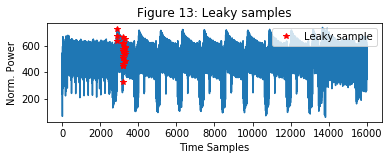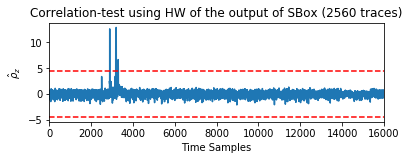The number of leaky point has been reduced to 23 points, since we are looking for time points (and operation on the device under test) involved in the computation of the S-box of the $1^{st}$ byte at the first round only.

In :
plt.figure(fignum)
plt.clf()
plt.subplot(211)
plt.plot(rho_normalized)
plt.plot([0, Nsamples-1],[4.5, 4.5], '--r')
plt.plot([0, Nsamples-1],[-4.5, -4.5], '--r')
plt.xlim(2200, 3500)
plt.ylabel(r'$\hat{\rho}_{z}$')
plt.title(r'Figure %.0f: Input-based $\rho$-test (upper) and HW-based $\rho$-test (lower)' % fignum)
plt.subplot(212)
plt.plot(rho_normalized_HW)
plt.plot([0, Nsamples-1],[4.5, 4.5], '--r')
plt.plot([0, Nsamples-1],[-4.5, -4.5], '--r')
plt.xlim(2200, 3500)
plt.xlabel('Time Samples')
plt.ylabel(r'$\hat{\rho}_{z}$')
plt.show()
fignum = fignum + 1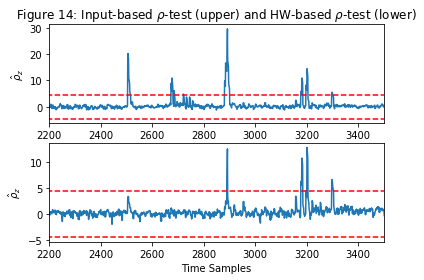Peaks observed in the input-based $\rho$-test are due to manipulation of useless plaintext byte and useful intermediate values, that depends bijectively on it (loading plaintext, XOR and S-box). Regarding the leaky peaks in the Hamming Weight based $\rho$-test, they are responsible for the power consumption related to the S-box computation only, and of course, their number is much smaller.

#### Authors:¶

D. Bellizia {davide.bellizia@uclouvain.be}, UniversitÃ© Catholique de Louvain, ICTEAM/Crypto Group

C. Whitnall {carolyn.whitnall@bristol.ac.uk}, University of Bristol, Dep. of Computer Science/Cryptographic Group

REASSURE (H2020 731591) http://reassure.eu/